### Example 27.22 Testing Equality of Covariance and Mean Matrices between Independent Groups

To make the specification of some standard MSTRUCT models for covariance and mean patterns more efficient, PROC CALIS defines these standard models internally. You can use two options to invoke these built-in covariance and mean patterns easily. For example, with the COVPATTERN= option, you can define the compound symmetry (COMPSYM) pattern for the covariance matrix or the equality of covariance matrices between groups (EQCOVMAT). With the MEANPATTERN= option, you can define uniform means (UNIFORM) for the mean vector or the equality of mean vectors between groups (EQMEANVEC). See the COVPATTERN= and the MEANPATTERN= options for details about the supported built-in covariance and mean patterns.

In Example 27.21, you test of the equality of covariance matrices between two groups. This example extends the application to the test of equality of mean vectors between three independent groups by using the COVPATTERN= and MEANPATTERN= options together. The best fit model for the data is explored. The following DATA steps define the covariance and mean matrices for the three independent groups, respectively:

```data g1(type=corr);
Input _type_ \$ 1-8 _name_ \$ 9-11 x1-x9;
datalines;
corr    x1  1.     .       .      .      .      .      .      .       .
corr    x2 .721    1.      .      .      .      .      .      .       .
corr    x3 .676   .379     1.     .      .      .      .      .       .
corr    x4 .149   .403    .450    1.     .      .      .      .       .
corr    x5 .422   .384    .445   .411    1.     .      .      .       .
corr    x6 .343   .456    .243   .308   .531    1.     .      .       .
corr    x7 .115   .225    .201   .481   .373   .198   1.      .       .
corr    x8 .213   .237    .434   .503   .267   .333   .355   1.       .
corr    x9 .236   .257    .159   .246   .126   .235   .601   .512    1.
mean     . 21.3   22.3    17.2   23.4   22.1   15.6   18.7   20.1  19.7
std      .  1.2    1.4    .87    1.33    2.2    1.4    2.3    2.1   1.8
n        .   21     21      21     21     21     21     21     21    21
;
```
```data g2(type=corr);
Input _type_ \$ 1-8 _name_ \$ 9-11 x1-x9;
datalines;
corr    x1  1.     .       .      .      .      .      .      .       .
corr    x2 .733    1.      .      .      .      .      .      .       .
corr    x3 .576   .388     1.     .      .      .      .      .       .
corr    x4 .209   .414    .425    1.     .      .      .      .       .
corr    x5 .412   .286    .461   .398    1.     .      .      .       .
corr    x6 .323   .399    .212   .302   .522    1.     .      .       .
corr    x7 .215   .295    .188   .467   .334   .232   1.      .       .
corr    x8 .204   .257    .462   .522   .298   .355  .372    1.       .
corr    x9 .245   .272    .177   .301   .156   .246  .578   .422     1.
mean     . 22.1   19.8    16.9   23.3   21.9   17.3   17.9   19.1  19.8
std      .  1.3    1.3    .99    1.25    2.1    1.3    2.2    2.0   1.5
n        .   22     22      22     22     22     22     22     22    22
;
```
```data g3(type=corr);
Input _type_ \$ 1-8 _name_ \$ 9-11 x1-x9;
datalines;
corr    x1  1.     .       .      .      .      .      .      .       .
corr    x2 .699    1.      .      .      .      .      .      .       .
corr    x3 .488   .328     1.     .      .      .      .      .       .
corr    x4 .235   .398    .413    1.     .      .      .      .       .
corr    x5 .377   .265    .471   .376    1.     .      .      .       .
corr    x6 .335   .412    .265   .314   .503    1.     .      .       .
corr    x7 .243   .216    .192   .423   .369   .212   1.      .       .
corr    x8 .217   .292    .423   .525   .219   .317  .376    1.       .
corr    x9 .211   .283    .152   .285   .147   .135  .633   .579     1.
mean     . 22.2   20.9    15.4   25.1   22.6   16.3   19.3   20.2  19.5
std      .  1.5    1.0    1.04    1.5    1.9    1.6    2.4    2.2   1.6
n        .   20     20      20     20     20     20     20     20    20
;
```

Each of these data sets contains the information about the correlations, means, standard deviations, and sample sizes. Even though these data sets contain correlations, by default PROC CALIS analyzes the covariances and means.

The first hypothesis to test is the equality of covariance matrices and mean vectors: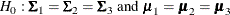where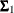,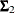, and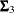are the population covariance matrices for the three independent groups, respectively, and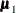,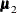, and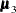are the population mean vectors for the three independent groups, respectively.

The following statements specify this test:

```proc calis covpattern=eqcovmat meanpattern=eqmeanvec;
var x1-x9;
group 1 / data=g1;
group 2 / data=g2;
group 3 / data=g3;
fitindex NoIndexType On(only)=[chisq df probchi rmsea aic caic sbc];
run;
```

In the PROC CALIS statement, the COVPATTERN=EQCOVMAT option specifies the same covariance matrix for the three groups and the MEANPATTERN=EQMEANVEC option specifies the same mean vector for the three groups. The VAR statement specifies that `x1``9` are the variables in the hypothesis test. Next, the GROUP statements specify the data sets for the three independent groups. You use the FITINDEX statement to limit the amount of output fit statistics to the quantities specified: the chi-square test (CHISQ), the degrees of freedom (DF), the significance value of the test statistic (PROBCHI), the root mean square error approximation (RMSEA), Akaike’s information criterion (AIC), consistent Akaike’s information criterion (CAIC), and Schwarz’s Bayesian criterion (SBC). The first three quantities are useful for the chi-square model fit test, while the rest of the fit indices are useful for comparing competing models for the data. Because there are not many quantities in this customized fit summary table, the NOINDEXTYPE option is used to suppress the printing of the fit index types.

Output 27.22.1 shows the general modeling information, including the sample sizes, the models for the groups, the model types, and the analysis types.

Output 27.22.1: Modeling Information for Testing Equality of Covariance and Mean Matrices

Modeling Information
Maximum Likelihood Estimation
Group Data Set N Obs Model Type Analysis
1 WORK.G1 21 Model 1 MSTRUCT Means and Covariances
2 WORK.G2 22 Model 2 MSTRUCT Means and Covariances
3 WORK.G3 20 Model 3 MSTRUCT Means and Covariances

Output 27.22.2 shows the initial mean vector and the initial covariance matrix specifications for Model 1, which fits to Group 1. PROC CALIS generates the mean parameter names `_mean_1`, `_mean_2`, …, and `_mean_9` for the nine elements in the mean vector. It also generates the covariance parameter names `_cov_1_1`, `_cov_2_1`, …, and `_cov_9_9` for the 45 nonredundant elements in the covariance matrix.

Output 27.22.2: Initial Mean Vector and Covariance Matrix for Model 1

Model 1. Initial MSTRUCT _MEAN_ Vector
Variable Parameter Estimate
x1 _mean_1 .
x2 _mean_2 .
x3 _mean_3 .
x4 _mean_4 .
x5 _mean_5 .
x6 _mean_6 .
x7 _mean_7 .
x8 _mean_8 .
x9 _mean_9 .

Model 1. Initial MSTRUCT _COV_ Matrix
x1 x2 x3 x4 x5 x6 x7 x8 x9
x1
 . [_cov_1_1]
 . [_cov_2_1]
 . [_cov_3_1]
 . [_cov_4_1]
 . [_cov_5_1]
 . [_cov_6_1]
 . [_cov_7_1]
 . [_cov_8_1]
 . [_cov_9_1]
x2
 . [_cov_2_1]
 . [_cov_2_2]
 . [_cov_3_2]
 . [_cov_4_2]
 . [_cov_5_2]
 . [_cov_6_2]
 . [_cov_7_2]
 . [_cov_8_2]
 . [_cov_9_2]
x3
 . [_cov_3_1]
 . [_cov_3_2]
 . [_cov_3_3]
 . [_cov_4_3]
 . [_cov_5_3]
 . [_cov_6_3]
 . [_cov_7_3]
 . [_cov_8_3]
 . [_cov_9_3]
x4
 . [_cov_4_1]
 . [_cov_4_2]
 . [_cov_4_3]
 . [_cov_4_4]
 . [_cov_5_4]
 . [_cov_6_4]
 . [_cov_7_4]
 . [_cov_8_4]
 . [_cov_9_4]
x5
 . [_cov_5_1]
 . [_cov_5_2]
 . [_cov_5_3]
 . [_cov_5_4]
 . [_cov_5_5]
 . [_cov_6_5]
 . [_cov_7_5]
 . [_cov_8_5]
 . [_cov_9_5]
x6
 . [_cov_6_1]
 . [_cov_6_2]
 . [_cov_6_3]
 . [_cov_6_4]
 . [_cov_6_5]
 . [_cov_6_6]
 . [_cov_7_6]
 . [_cov_8_6]
 . [_cov_9_6]
x7
 . [_cov_7_1]
 . [_cov_7_2]
 . [_cov_7_3]
 . [_cov_7_4]
 . [_cov_7_5]
 . [_cov_7_6]
 . [_cov_7_7]
 . [_cov_8_7]
 . [_cov_9_7]
x8
 . [_cov_8_1]
 . [_cov_8_2]
 . [_cov_8_3]
 . [_cov_8_4]
 . [_cov_8_5]
 . [_cov_8_6]
 . [_cov_8_7]
 . [_cov_8_8]
 . [_cov_9_8]
x9
 . [_cov_9_1]
 . [_cov_9_2]
 . [_cov_9_3]
 . [_cov_9_4]
 . [_cov_9_5]
 . [_cov_9_6]
 . [_cov_9_7]
 . [_cov_9_8]
 . [_cov_9_9]

Although not shown here, the initial mean vector and covariance matrices for Models 2 and 3 are exactly the same as those shown in Output 27.22.2, as required by the equality of covariance and mean matrices in the null hypothesis.

Output 27.22.3 shows the customized fit summary table. The chi-square test statistic is 203.2605. The degrees of freedom is 108 and the p-value is less than 0.0001. Therefore, the hypothesisof equality in covariance and mean matrices is rejected for the three independent groups. The RMSEA index is much greater than 0.05, which does not indicate a good model fit. Other fit indices such as AIC, CAIC, and SBC are not interpreted for the fit of the model itself, but are useful for comparing competing models in the later discussion.

Output 27.22.3: Fit Summary for Testing: Equality of Covariance and Mean Matrices

Fit Summary
Chi-Square 203.2605
Chi-Square DF 108
Pr > Chi-Square <.0001
RMSEA Estimate 0.2100
Akaike Information Criterion 311.2605
Bozdogan CAIC 480.9897
Schwarz Bayesian Criterion 426.9897

A less restrictive hypothesis is now considered. This hypothesis states the equality of covariance matrices only: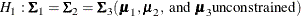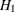differs fromin that the population means inare not constrained. To test this hypothesis, you need to change the MEANPATTERN= option to use the SATURATED keyword, as shown in the following statements:

```proc calis covpattern=eqcovmat meanpattern=saturated;
var x1-x9;
group 1 / data=g1;
group 2 / data=g2;
group 3 / data=g3;
fitindex NoIndexType On(only)=[chisq df probchi rmsea aic caic sbc];
run;
```

Output 27.22.4 shows the results of the testing.

Output 27.22.4: Fit Summary for Testing: Equality of Covariance Matrices but Unconstrained Means

Fit Summary
Chi-Square 26.7897
Chi-Square DF 90
Pr > Chi-Square 1.0000
RMSEA Estimate 0.0000
Akaike Information Criterion 170.7897
Bozdogan CAIC 397.0954
Schwarz Bayesian Criterion 325.0954

The chi-square test statistic is 26.7897 (df = 90, p = 1.000). You cannot reject this null hypothesis about the equality of the population covariance matrices. The RMSEA value is virtually zero, which indicates a perfect fit. Comparing the models underand, it is clear that the three groups are significantly different with regard to their mean vectors. By relaxing all the equality constraints on the means in,is derived and is supported by the chi-square test. In addition, the RMSEA value for the model underis perfect. Because lower values of AIC, CAIC, and SBC values indicate better model fit (with the model complexity taken into account), these indices in Output 27.22.3 and Output 27.22.4 support that the model underis better than.

However, in getting a superior model fit,might have relaxed more constraints than absolutely necessary for an optimal fit. That is, it might be possible to impose equality constraints on only some (but not all, as in) of the means to reach the same or even better model fit (by the RMSEA, AIC, CAIC, or SBC criterion) than the model under. But how can you determine this set of constrained means?

To answer this question, you conduct an exploratory analysis of the data by using some model modification techniques. Models established from exploratory analysis should be validated by external data in the future. However, this example demonstrates the exploratory part only.

Beginning with the model under, you can manually take away some particular constraints on the means and explore whether the revised model improves the fit. If the revised model fits better, you can repeat the process until you cannot improve more. Ultimately, you might be able to find the best model between the models specified underand. Such an exploratory analysis is laborious, considering the vast possibilities of constraints on the nine variable means in three independent groups that you could attempt to release. Fortunately, PROC CALIS provides some model modification statistics, called the LM (Lagrange multiplier) statistics, to assist this kind of exploratory analysis.

The following statements specify the model under, but now with the MODIFICATION option added to the PROC CALIS statement:

```proc calis covpattern=eqcovmat meanpattern=eqmeanvec modification;
var x1-x9;
group 1 / data=g1;
group 2 / data=g2;
group 3 / data=g3;
fitindex NoIndexType On(only)=[chisq df probchi rmsea aic caic sbc];
run;
```

The MODIFICATION option requests the so-called LM (Lagrange multiplier) statistics for releasing the parameter constraints. These constraints include the cross-group or within-group constraints and the fixed values in the model. For the model under, the covariances and the means are all constrained across groups. These are the equality constraints that you would like to release to obtain a better model fit. Output 27.22.5 shows the results of the LM statistics for releasing these equality constraints in variances, covariances, and means.

Output 27.22.5: Lagrange Multiplier Statistics for Releasing the Equality Constraints

Lagrange Multiplier Statistics for Releasing Equality Constraints
Parm Released Parameter LM Stat Pr > ChiSq Changes

Model

Type
Var1 Var2 Original
Parm
Released
Parm
_cov_1_1 1 COV x1 x1 0.01137 0.9151 0.0178 -0.0355
2 COV x1 x1 1.00150 0.3169 0.1729 -0.3212
3 COV x1 x1 1.28632 0.2567 -0.1818 0.3923
_cov_2_1 1 COV x2 x1 2.19353 0.1386 0.2038 -0.4076
2 COV x2 x1 0.77014 0.3802 -0.1253 0.2327
3 COV x2 x1 0.36128 0.5478 -0.0796 0.1718
_cov_2_2 1 COV x2 x2 3.12065 0.0773 -0.4344 0.8687
2 COV x2 x2 0.05704 0.8112 -0.0609 0.1132
3 COV x2 x2 4.14151 0.0418 0.4817 -1.0395
_cov_3_1 1 COV x3 x1 0.00672 0.9347 0.00888 -0.0178
2 COV x3 x1 2.23758 0.1347 -0.1681 0.3122
3 COV x3 x1 2.10455 0.1469 0.1512 -0.3264
_cov_3_2 1 COV x3 x2 2.18538 0.1393 -0.1940 0.3881
2 COV x3 x2 3.14532 0.0761 0.2416 -0.4487
3 COV x3 x2 0.10264 0.7487 -0.0405 0.0874
_cov_3_3 1 COV x3 x3 1.56813 0.2105 0.1815 -0.3630
2 COV x3 x3 0.66118 0.4161 0.1223 -0.2272
3 COV x3 x3 4.42160 0.0355 -0.2934 0.6332
_cov_4_1 1 COV x4 x1 0.31691 0.5735 -0.0667 0.1333
2 COV x4 x1 0.32615 0.5679 0.0702 -0.1304
3 COV x4 x1 0.0002277 0.9880 -0.00172 0.00371
_cov_4_2 1 COV x4 x2 0.73377 0.3917 0.1242 -0.2484
2 COV x4 x2 0.53196 0.4658 -0.1097 0.2038
3 COV x4 x2 0.01445 0.9043 -0.0168 0.0362
_cov_4_3 1 COV x4 x3 0.0000258 0.9959 0.000547 -0.00109
2 COV x4 x3 0.24892 0.6178 -0.0558 0.1036
3 COV x4 x3 0.25646 0.6126 0.0525 -0.1134
_cov_4_4 1 COV x4 x4 0.04412 0.8336 0.0361 -0.0722
2 COV x4 x4 0.52198 0.4700 0.1288 -0.2392
3 COV x4 x4 0.90948 0.3403 -0.1577 0.3403
_cov_5_1 1 COV x5 x1 0.0008607 0.9766 -0.00477 0.00953
2 COV x5 x1 0.01238 0.9114 0.0188 -0.0348
3 COV x5 x1 0.00712 0.9328 -0.0132 0.0285
_cov_5_2 1 COV x5 x2 0.10637 0.7443 -0.0649 0.1297
2 COV x5 x2 0.00631 0.9367 -0.0164 0.0304
3 COV x5 x2 0.16971 0.6804 0.0789 -0.1702
_cov_5_3 1 COV x5 x3 0.06645 0.7966 -0.0385 0.0771
2 COV x5 x3 0.0008275 0.9771 0.00446 -0.00829
3 COV x5 x3 0.05370 0.8167 0.0334 -0.0720
_cov_5_4 1 COV x5 x4 0.24212 0.6227 0.0809 -0.1617
2 COV x5 x4 0.04459 0.8328 -0.0360 0.0669
3 COV x5 x4 0.07959 0.7779 -0.0446 0.0963
_cov_5_5 1 COV x5 x5 0.01778 0.8939 -0.0431 0.0862
2 COV x5 x5 0.08223 0.7743 -0.0962 0.1787
3 COV x5 x5 0.18417 0.6678 0.1336 -0.2883
_cov_6_1 1 COV x6 x1 0.29558 0.5867 -0.0721 0.1442
2 COV x6 x1 0.26589 0.6061 -0.0710 0.1318
3 COV x6 x1 1.16570 0.2803 0.1378 -0.2974
_cov_6_2 1 COV x6 x2 0.00228 0.9619 -0.00780 0.0156
2 COV x6 x2 1.00319 0.3165 0.1697 -0.3152
3 COV x6 x2 0.95767 0.3278 -0.1538 0.3320
_cov_6_3 1 COV x6 x3 1.39116 0.2382 0.1513 -0.3027
2 COV x6 x3 0.08741 0.7675 -0.0394 0.0731
3 COV x6 x3 0.79586 0.3723 -0.1102 0.2378
_cov_6_4 1 COV x6 x4 0.46031 0.4975 -0.0947 0.1894
2 COV x6 x4 0.04254 0.8366 0.0299 -0.0555
3 COV x6 x4 0.22665 0.6340 0.0640 -0.1381
_cov_6_5 1 COV x6 x5 0.14991 0.6986 -0.0700 0.1399
2 COV x6 x5 0.04723 0.8280 0.0408 -0.0757
3 COV x6 x5 0.02874 0.8654 0.0295 -0.0636
_cov_6_6 1 COV x6 x6 0.22550 0.6349 0.1079 -0.2158
2 COV x6 x6 0.04390 0.8340 0.0494 -0.0918
3 COV x6 x6 0.48451 0.4864 -0.1523 0.3286
_cov_7_1 1 COV x7 x1 0.50774 0.4761 0.1203 -0.2406
2 COV x7 x1 0.01246 0.9111 -0.0196 0.0363
3 COV x7 x1 0.36926 0.5434 -0.0988 0.2131
_cov_7_2 1 COV x7 x2 0.01235 0.9115 0.0228 -0.0455
2 COV x7 x2 0.16400 0.6855 -0.0861 0.1598
3 COV x7 x2 0.09159 0.7622 0.0597 -0.1288
_cov_7_3 1 COV x7 x3 0.16844 0.6815 -0.0644 0.1288
2 COV x7 x3 0.15095 0.6976 0.0633 -0.1175
3 COV x7 x3 0.0003079 0.9860 0.00265 -0.00572
_cov_7_4 1 COV x7 x4 0.22542 0.6349 -0.0776 0.1551
2 COV x7 x4 0.00754 0.9308 0.0147 -0.0273
3 COV x7 x4 0.15376 0.6950 0.0617 -0.1331
_cov_7_5 1 COV x7 x5 0.07831 0.7796 -0.0631 0.1262
2 COV x7 x5 0.07552 0.7835 0.0643 -0.1195
3 COV x7 x5 3.293E-6 0.9986 0.000394 -0.00085
_cov_7_6 1 COV x7 x6 0.13810 0.7102 0.0726 -0.1452
2 COV x7 x6 0.0001086 0.9917 0.00211 -0.00392
3 COV x7 x6 0.14999 0.6985 -0.0729 0.1572
_cov_7_7 1 COV x7 x7 0.09334 0.7600 0.1051 -0.2101
2 COV x7 x7 0.00128 0.9714 0.0128 -0.0237
3 COV x7 x7 0.11994 0.7291 -0.1147 0.2474
_cov_8_1 1 COV x8 x1 0.04800 0.8266 0.0353 -0.0706
2 COV x8 x1 0.19725 0.6569 0.0743 -0.1379
3 COV x8 x1 0.45888 0.4981 -0.1051 0.2268
_cov_8_2 1 COV x8 x2 0.13689 0.7114 0.0727 -0.1453
2 COV x8 x2 0.31671 0.5736 -0.1147 0.2130
3 COV x8 x2 0.04084 0.8398 0.0382 -0.0825
_cov_8_3 1 COV x8 x3 0.37615 0.5397 -0.0904 0.1808
2 COV x8 x3 0.00452 0.9464 -0.0103 0.0191
3 COV x8 x3 0.47678 0.4899 0.0980 -0.2114
_cov_8_4 1 COV x8 x4 0.00989 0.9208 0.0150 -0.0300
2 COV x8 x4 0.01001 0.9203 0.0157 -0.0291
3 COV x8 x4 0.04138 0.8388 -0.0296 0.0638
_cov_8_5 1 COV x8 x5 0.01378 0.9066 -0.0267 0.0533
2 COV x8 x5 0.03154 0.8590 -0.0419 0.0778
3 COV x8 x5 0.09063 0.7634 0.0659 -0.1421
_cov_8_6 1 COV x8 x6 0.0007193 0.9786 0.00510 -0.0102
2 COV x8 x6 0.01293 0.9095 0.0224 -0.0417
3 COV x8 x6 0.02067 0.8857 -0.0263 0.0568
_cov_8_7 1 COV x8 x7 0.16543 0.6842 0.0952 -0.1904
2 COV x8 x7 0.29902 0.5845 -0.1328 0.2467
3 COV x8 x7 0.02206 0.8819 0.0335 -0.0722
_cov_8_8 1 COV x8 x8 0.00581 0.9392 0.0244 -0.0487
2 COV x8 x8 0.00694 0.9336 -0.0276 0.0513
3 COV x8 x8 0.0000660 0.9935 0.00250 -0.00539
_cov_9_1 1 COV x9 x1 0.19272 0.6607 -0.0532 0.1063
2 COV x9 x1 0.01910 0.8901 -0.0174 0.0323
3 COV x9 x1 0.34408 0.5575 0.0684 -0.1476
_cov_9_2 1 COV x9 x2 0.09017 0.7640 -0.0446 0.0892
2 COV x9 x2 0.26496 0.6067 0.0794 -0.1474
3 COV x9 x2 0.04994 0.8232 -0.0320 0.0690
_cov_9_3 1 COV x9 x3 0.44236 0.5060 0.0758 -0.1516
2 COV x9 x3 0.12761 0.7209 -0.0422 0.0784
3 COV x9 x3 0.09470 0.7583 -0.0338 0.0728
_cov_9_4 1 COV x9 x4 0.04619 0.8298 0.0260 -0.0520
2 COV x9 x4 0.22996 0.6316 -0.0602 0.1117
3 COV x9 x4 0.07502 0.7842 0.0319 -0.0688
_cov_9_5 1 COV x9 x5 0.02807 0.8669 0.0279 -0.0557
2 COV x9 x5 0.0006585 0.9795 -0.00443 0.00823
3 COV x9 x5 0.02058 0.8859 -0.0230 0.0496
_cov_9_6 1 COV x9 x6 0.03989 0.8417 -0.0282 0.0563
2 COV x9 x6 0.15069 0.6979 -0.0568 0.1055
3 COV x9 x6 0.36051 0.5482 0.0815 -0.1759
_cov_9_7 1 COV x9 x7 0.03398 0.8537 -0.0284 0.0567
2 COV x9 x7 0.05802 0.8097 0.0385 -0.0714
3 COV x9 x7 0.00362 0.9520 -0.00891 0.0192
_cov_9_8 1 COV x9 x8 0.06050 0.8057 -0.0391 0.0781
2 COV x9 x8 0.56151 0.4537 0.1235 -0.2294
3 COV x9 x8 0.26945 0.6037 -0.0794 0.1713
_cov_9_9 1 COV x9 x9 0.13296 0.7154 -0.0655 0.1310
2 COV x9 x9 0.00130 0.9712 -0.00673 0.0125
3 COV x9 x9 0.16526 0.6844 0.0703 -0.1517
_mean_1 1 MEAN x1   11.09173 0.0009 0.3453 -0.6906
2 MEAN x1   1.21196 0.2709 -0.1184 0.2200
3 MEAN x1   5.04550 0.0247 -0.2242 0.4838
_mean_2 1 MEAN x2   21.46921 <.0001 -0.5837 1.1675
2 MEAN x2   15.27776 <.0001 0.5110 -0.9490
3 MEAN x2   0.47301 0.4916 0.0834 -0.1800
_mean_3 1 MEAN x3   4.41967 0.0355 -0.2034 0.4067
2 MEAN x3   6.37770 0.0116 -0.2535 0.4708
3 MEAN x3   22.27732 <.0001 0.4395 -0.9485
_mean_4 1 MEAN x4   3.26860 0.0706 0.1904 -0.3807
2 MEAN x4   0.03260 0.8567 0.0197 -0.0366
3 MEAN x4   4.06935 0.0437 -0.2045 0.4413
_mean_5 1 MEAN x5   0.22210 0.6374 -0.0681 0.1362
2 MEAN x5   1.50172 0.2204 0.1837 -0.3412
3 MEAN x5   0.60673 0.4360 -0.1083 0.2338
_mean_6 1 MEAN x6   1.61486 0.2038 0.1539 -0.3078
2 MEAN x6   6.72912 0.0095 -0.3260 0.6055
3 MEAN x6   1.88248 0.1701 0.1600 -0.3452
_mean_7 1 MEAN x7   0.14035 0.7079 -0.0558 0.1116
2 MEAN x7   0.11034 0.7398 0.0514 -0.0954
3 MEAN x7   0.00153 0.9688 0.00560 -0.0121
_mean_8 1 MEAN x8   0.12603 0.7226 -0.0510 0.1019
2 MEAN x8   1.96607 0.1609 0.2089 -0.3880
3 MEAN x8   1.16200 0.2811 -0.1490 0.3215
_mean_9 1 MEAN x9   0.05301 0.8179 0.0248 -0.0496
2 MEAN x9   0.97965 0.3223 -0.1106 0.2054
3 MEAN x9   0.61083 0.4345 0.0810 -0.1748

To use the results of this table, you look for parameters that have large LM statistics (in the LM Stat column). Equivalently, you can look for parameters that have small p-values (in the Pr > ChiSq column). Loosely speaking, an LM statistic estimates the reduction of model fit chi-square statistic if you release the constraint on the corresponding parameter. The p-value indicates whether the improvement would be significant. Therefore, releasing those parameters with a high LM statistic and small p-value would be the key to model improvements. Bear in mind that the LM statistics are linear approximations and they might not be very accurate as estimates of the actual model improvement, which could only be accessed when you refit the model with the particular constraint released. Nonetheless, the LM statistics could still be very useful because they show which constraints could potentially improve the model the most.

Output 27.22.5 shows the results from releasing the constraints on the variances and covariances first. Each constrained element of the covariance matrix has three rows, respectively, for the three models (or groups). For example, the first parameter is `_cov_1_1`, which is the same variance parameter for `x1` in the three models. The first row shows that if you release the variance of `x1` in Model 1 from the constraint (while keeping the variances of `x1` being constrained between Models 2 and 3), the LM statistic is 0.01127, and the corresponding p-value is 0.9155. This means that the model fit improvement would be very small and so you do not expect a significant model fit improvement by releasing this constraint. The columns entitled Changes show the estimated parameter changes in the original parameters (that is, `_cov_1_1` for Models 2 and 3) and in the released parameter (that is, the new parameter for the variance of `x1` in Model 1) if you release the corresponding equality constraint. These two Changes columns are not very useful for the present purpose.

Looking through the results for the variance and covariance constraints, you can see that almost all the associated p-values are large (that is, as compared with the conventional 0.05 level for significance). Therefore, all these constraints on variances and covariances would not improve the model fit significantly. In contrast, the constraints on the means show that several of them could be released for a sizable model fit improvement. The largest LM statistic in the table is the one for `_mean_3` in Model 3. The LM statistic is 22.27678 and its corresponding p-value is less than 0.0001. This means that if the mean of `x3` in Model 3 were not constrained with the means of `x3` in Models 1 and 2, you would have expected a reduction in the model fit chi-square statistic that is estimated at 22.27678. Other notable LM statistics are those for `_mean_1` in Model 1, `_mean_2` in Model 1 or 2, and `_mean_6` in Model 2.

Two important points are noted about the use of the LM statistics. First, the LM statistics are not additive. You cannot expect that the total reduction in model fit chi-square for releasing a particular set of parameter constraints is the sum of the corresponding LM statistics. Second, once you release a particular constraint and refit the model, the LM statistics in the revised model might not follow the same pattern as those LM statistics in the original model. Basically, these are due to the nonlinearity of the fit function and the dependence of the parameter estimates. Therefore, in order to find the best model for the data, it would be more sensible to adopt a one-at-a-time approach to release the constraints. That is, you release one constraint at a time and refit the model to see if you can release more constraints to improve the model fit.

According to the results of LM statistics in Output 27.22.5, you first release the constraint on the `_mean_3` parameter, which is for the mean of `x3` in Model 3. The following statements fit such a model:

```proc calis modification;
var x1-x9;
group 1 / data=g1;
group 2 / data=g2;
group 3 / data=g3;
model 1 / group = 1;
mstruct;
matrix _cov_  = cov01-cov45;
matrix _mean_ = mean1-mean9;
model 2 / group = 2;
refmodel 1;
model 3 / group = 3;
refmodel 1;
renameparm mean3=mean3_mdl3;
fitindex NoIndexType On(only)=[chisq df probchi rmsea aic caic sbc];
run;
```

Because the revised model is no longer a supported built-in MSTRUCT model, you cannot use the MEANPATTERN= or the COVPATTERN= options any more. Instead, you now use the MSTRUCT modeling language to specify the covariance and mean patterns. Model 1, which fits to Group 1, is an MSTRUCT model with variance and covariance parameters `cov01``cov45` and mean parameters `mean1``mean9`. Model 2, which fits to Group 2, refers to the specifications of Model 1, as indicated in a REFMODEL statement. Hence, Model 1 and Model 2 are completely constrained in variances, covariances, and means. Model 3, which fits to Group 3, also refers to the specifications of Model 1, as indicated in another REFMODEL statement. However, the RENAMEPARM statement renames the parameter `mean3` in the reference model (that is, Model 1) to a new name `mean3_mdl3`. As a results, all variance, covariance, and mean parameters except one in Model 3 are constrained to be the same as those in Model 1. The mean of `x3` in Model 3 is the only parameter that is not constrained with any other parameters. This forms the first revised model from. The MODIFICATION option is specified again to determine whether a further model fit improvement is possible.

Output 27.22.6 shows the modeling information of the first revised model. It shows that Models 2 and 3 make references to Model 1. Therefore, parameters between models are constrained by referencing.

Output 27.22.6: Modeling Information for The First Revised Model

Modeling Information
Maximum Likelihood Estimation
Group Data Set N Obs Model Type Base Model Analysis
1 WORK.G1 21 Model 1 MSTRUCT   Means and Covariances
2 WORK.G2 22 Model 2 MSTRUCT Model 1 Means and Covariances
3 WORK.G3 20 Model 3 MSTRUCT Model 1 Means and Covariances

Output 27.22.7 shows the initial specifications of the means, variances, and covariances in Model 1.

Output 27.22.7: Initial Mean Vector and Covariance Matrix for Model 1 in the First Revised Model

Model 1. Initial MSTRUCT _MEAN_ Vector
Variable Parameter Estimate
x1 mean1 .
x2 mean2 .
x3 mean3 .
x4 mean4 .
x5 mean5 .
x6 mean6 .
x7 mean7 .
x8 mean8 .
x9 mean9 .

Model 1. Initial MSTRUCT _COV_ Matrix
x1 x2 x3 x4 x5 x6 x7 x8 x9
x1
 . [cov01]
 . [cov02]
 . [cov04]
 . [cov07]
 . [cov11]
 . [cov16]
 . [cov22]
 . [cov29]
 . [cov37]
x2
 . [cov02]
 . [cov03]
 . [cov05]
 . [cov08]
 . [cov12]
 . [cov17]
 . [cov23]
 . [cov30]
 . [cov38]
x3
 . [cov04]
 . [cov05]
 . [cov06]
 . [cov09]
 . [cov13]
 . [cov18]
 . [cov24]
 . [cov31]
 . [cov39]
x4
 . [cov07]
 . [cov08]
 . [cov09]
 . [cov10]
 . [cov14]
 . [cov19]
 . [cov25]
 . [cov32]
 . [cov40]
x5
 . [cov11]
 . [cov12]
 . [cov13]
 . [cov14]
 . [cov15]
 . [cov20]
 . [cov26]
 . [cov33]
 . [cov41]
x6
 . [cov16]
 . [cov17]
 . [cov18]
 . [cov19]
 . [cov20]
 . [cov21]
 . [cov27]
 . [cov34]
 . [cov42]
x7
 . [cov22]
 . [cov23]
 . [cov24]
 . [cov25]
 . [cov26]
 . [cov27]
 . [cov28]
 . [cov35]
 . [cov43]
x8
 . [cov29]
 . [cov30]
 . [cov31]
 . [cov32]
 . [cov33]
 . [cov34]
 . [cov35]
 . [cov36]
 . [cov44]
x9
 . [cov37]
 . [cov38]
 . [cov39]
 . [cov40]
 . [cov41]
 . [cov42]
 . [cov43]
 . [cov44]
 . [cov45]

Output 27.22.8 shows the initial specifications of the means in Model 2. The mean parameters in Model 2 are exactly the same as those in Model 1, as shown in Output 27.22.7. The variance and covariance parameters in Model 2 are also exactly the same as those in Model 1, but are not shown here to conserve space.

Output 27.22.8: Initial Mean Vector for Model 2 in the First Revised Model

Model 2. Initial MSTRUCT _MEAN_ Vector
Variable Parameter Estimate
x1 mean1 .
x2 mean2 .
x3 mean3 .
x4 mean4 .
x5 mean5 .
x6 mean6 .
x7 mean7 .
x8 mean8 .
x9 mean9 .

Output 27.22.9 shows the initial specifications of the means in Model 3. All but one mean parameter in Model 3 are exactly the same as those in Models 1 and 2, as shown in Output 27.22.7 and Output 27.22.8, respectively. The mean for `x3` in Model 3 is `mean3_mdl3`, which is now a distinct parameter, and therefore it is not constrained with any other parameters in the first or the second models for Groups 1 or 2. However, the variance and covariance parameters in Model 3 are exactly the same as those in Model 1. They are not shown here to conserve space.

Output 27.22.9: Initial Mean Vector for Model 3 in the First Revised Model

Model 3. Initial MSTRUCT _MEAN_ Vector
Variable Parameter Estimate
x1 mean1 .
x2 mean2 .
x3 mean3_mdl3 .
x4 mean4 .
x5 mean5 .
x6 mean6 .
x7 mean7 .
x8 mean8 .
x9 mean9 .

Output 27.22.10 shows the fit summary of the first revised model. The model fit chi-square is 148.8865, which drops quite a bit from the original model under. The p-value of the model fit chi-square is 0.0046, which is statistically significant. The RMSEA value is 0.1399, which is also a sizable improvement. All the AIC, CAIC, and SBC values are reduced, indicating better model fit than the model under.

Output 27.22.10: Fit Summary for the First Revised Model

Fit Summary
Chi-Square 148.8865
Chi-Square DF 107
Pr > Chi-Square 0.0046
RMSEA Estimate 0.1399
Akaike Information Criterion 258.8865
Bozdogan CAIC 431.7589
Schwarz Bayesian Criterion 376.7589

Output 27.22.11 shows the LM statistics for releasing the equality constraints in the first revised model. Almost all of the results for the variance and covariance constraints are omitted because their LM statistics are not significant. However, Output 27.22.11 shows all the LM statistics for releasing the constraints in means. The mean of `x2` in Model 2 has the largest LM statistic at 26.25044.

Output 27.22.11: LM Statistics for Releasing the Equality Constraints in the First Revised Model

Lagrange Multiplier Statistics for Releasing Equality Constraints
Parm Released Parameter LM Stat Pr > ChiSq Changes

Model
Type Var1 Var2 Original
Parm
Released
Parm
cov01 1 COV x1 x1 0.64999 0.4201 0.1050 -0.2100
2 COV x1 x1 0.41758 0.5181 0.0874 -0.1622
3 COV x1 x1 2.18923 0.1390 -0.1855 0.4004
.
.
.
mean1 1 MEAN x1   9.26674 0.0023 0.2872 -0.5745
2 MEAN x1   3.00599 0.0830 -0.1702 0.3160
3 MEAN x1   2.13787 0.1437 -0.1481 0.3196
mean2 1 MEAN x2   26.25115 <.0001 -0.6568 1.3135
2 MEAN x2   12.34638 0.0004 0.4674 -0.8680
3 MEAN x2   2.52683 0.1119 0.1962 -0.4234
mean3 1 MEAN x3   0.58891 0.4428 -0.0787 0.0827
2 MEAN x3   0.58891 0.4428 0.0827 -0.0787
mean4 1 MEAN x4   6.59009 0.0103 0.2746 -0.5493
2 MEAN x4   0.51343 0.4737 0.0796 -0.1478
3 MEAN x4   11.61610 0.0007 -0.3586 0.7739
mean5 1 MEAN x5   0.52967 0.4667 -0.1042 0.2084
2 MEAN x5   0.22294 0.6368 0.0702 -0.1304
3 MEAN x5   0.06889 0.7930 0.0374 -0.0807
mean6 1 MEAN x6   1.16656 0.2801 0.1270 -0.2540
2 MEAN x6   5.29599 0.0214 -0.2810 0.5218
3 MEAN x6   1.69412 0.1931 0.1518 -0.3275
mean7 1 MEAN x7   0.03791 0.8456 -0.0291 0.0582
2 MEAN x7   0.44510 0.5047 0.1036 -0.1923
3 MEAN x7   0.23804 0.6256 -0.0704 0.1520
mean8 1 MEAN x8   0.39420 0.5301 -0.0883 0.1765
2 MEAN x8   0.24231 0.6225 0.0719 -0.1335
3 MEAN x8   0.01951 0.8889 0.0200 -0.0431
mean9 1 MEAN x9   0.00156 0.9685 0.00423 -0.00846
2 MEAN x9   1.06866 0.3012 -0.1150 0.2136
3 MEAN x9   1.05210 0.3050 0.1065 -0.2297

You now modify the preceding statements to specify the second revised model, as shown in the following statements:

```proc calis modification;
var x1-x9;
group 1 / data=g1;
group 2 / data=g2;
group 3 / data=g3;
model 1 / group = 1;
mstruct;
matrix _cov_  = cov01-cov45;
matrix _mean_ = mean1-mean9;
model 2 / group = 2;
refmodel 1;
renameparm mean2=mean2_new;    /* constraint a */
model 3 / group = 3;
refmodel 1;
renameparm mean2=mean2_new,    /* constraint a */
mean3=mean3_mdl3;
fitindex NoIndexType On(only)=[chisq df probchi rmsea aic caic sbc];
run;
```

This second revised model must not constrain the mean of `x2` in Model 1 with any parameters. A straightforward way to do this is to rename the `mean2` parameter to a unique name in Model 1. However, for the current specification it is more convenient to rename the `mean2` parameter in Models 2 and 3 to another name. In the specification of the second revised model, Models 2 and 3 still make references to Model 1. However, in the respective RENAMEPARM statements, both Model 2 and 3 rename the `mean2` parameter that is referenced from Model 1 to the new name `mean2_new`. This way the mean for `x2` in Model 1 is not constrained with the means of `x2` in Models 2 and 3. But the means for `x2` in Models 2 and 3 are still constrained to be equal by the same parameter `mean2_new`. Output 27.22.12 shows the fit summary of the second revised model.

Output 27.22.12: Fit Summary for the Second Revised Model

Fit Summary
Chi-Square 86.3927
Chi-Square DF 106
Pr > Chi-Square 0.9183
RMSEA Estimate 0.0000
Akaike Information Criterion 198.3927
Bozdogan CAIC 374.4083
Schwarz Bayesian Criterion 318.4083

Again, a sizable improvement over the first revised model is shown in the second revised model. The model fit chi-square statistic is no longer significant (p = 0.9183), and the RMSEA value is perfect at 0. Large drops in the AIC, CAIC, and SBC values are also observed.

Output 27.22.13 suggests that the mean of `x6` in Model 2 (which has the largest LM statistic at 11.41243) could be released from the equality constraints to achieve the largest model improvement over the current model.

Output 27.22.13: LM Statistics for Releasing the Equality Constraints in the Second Revised Model

Lagrange Multiplier Statistics for Releasing Equality Constraints
Parm Released Parameter LM Stat Pr > ChiSq Changes

Model
Type Var1 Var2 Original
Parm
Released
Parm
cov01 1 COV x1 x1 2.77024 0.0960 0.1384 -0.2770
2 COV x1 x1 0.28728 0.5920 0.0462 -0.0860
3 COV x1 x1 5.00087 0.0253 -0.1791 0.3864
.
.
.
mean1 1 MEAN x1   2.75437 0.0970 0.1646 -0.3292
2 MEAN x1   3.21093 0.0731 -0.1511 0.2806
3 MEAN x1   0.24923 0.6176 0.0424 -0.0915
mean3 1 MEAN x3   0.74338 0.3886 -0.0877 0.0934
2 MEAN x3   0.74338 0.3886 0.0934 -0.0877
mean4 1 MEAN x4   6.17449 0.0130 0.2672 -0.5343
2 MEAN x4   0.02087 0.8851 -0.0146 0.0272
3 MEAN x4   4.71344 0.0299 -0.2072 0.4470
mean5 1 MEAN x5   1.65517 0.1983 -0.1853 0.3706
2 MEAN x5   1.16118 0.2812 0.1606 -0.2982
3 MEAN x5   0.04040 0.8407 0.0287 -0.0618
mean6 1 MEAN x6   5.03834 0.0248 0.2712 -0.5423
2 MEAN x6   11.41247 0.0007 -0.4217 0.7831
3 MEAN x6   1.51175 0.2189 0.1460 -0.3150
mean7 1 MEAN x7   0.32382 0.5693 -0.0853 0.1706
2 MEAN x7   0.82184 0.3646 0.1410 -0.2619
3 MEAN x7   0.12513 0.7235 -0.0512 0.1104
mean8 1 MEAN x8   2.39207 0.1220 -0.2210 0.4420
2 MEAN x8   1.58292 0.2083 0.1867 -0.3467
3 MEAN x8   0.08641 0.7688 0.0427 -0.0922
mean9 1 MEAN x9   0.00682 0.9342 0.00886 -0.0177
2 MEAN x9   1.20949 0.2714 -0.1225 0.2274
3 MEAN x9   1.10016 0.2942 0.1089 -0.2349
mean2_new 2 MEAN x2   4.47814 0.0343 0.2983 -0.2661
3 MEAN x2   4.47814 0.0343 -0.2661 0.2983

The process of model refitting should now become familiar. You modify the previous model to release the constraint on the mean of `x6` in Model 2. As a result, the third revised model is specified by the following statements:

```proc calis modification;
var x1-x9;
group 1 / data=g1;
group 2 / data=g2;
group 3 / data=g3;
model 1 / group = 1;
mstruct;
matrix _cov_  = cov01-cov45;
matrix _mean_ = mean1-mean9;
model 2 / group = 2;
refmodel 1;
renameparm mean2=mean2_new,     /* constraint a */
mean6=mean6_mdl2;
model 3 / group = 3;
refmodel 1;
renameparm mean2=mean2_new,     /* constraint a */
mean3=mean3_mdl3;
fitindex NoIndexType On(only)=[chisq df probchi rmsea aic caic sbc];
run;
```

The only modification from the previous specification is to rename `mean6` to `mean6_mdl2` in the RENAMEPARM statement of Model 2. Output 27.22.14 shows the model fit summary of the third revised model.

Output 27.22.14: Fit Summary for the Third Revised Model

Fit Summary
Chi-Square 68.7869
Chi-Square DF 105
Pr > Chi-Square 0.9976
RMSEA Estimate 0.0000
Akaike Information Criterion 182.7869
Bozdogan CAIC 361.9456
Schwarz Bayesian Criterion 304.9456

The model improvement over the second revised model is still notable in the third revised model. The chi-square value drops about 20 points in the third revised model. The AIC, CAIC, and the SBC values are reduced notably, though not as impressively as with the previous improvements.

Output 27.22.15 suggests that the mean of `x4` in Model 1 (which has the largest LM statistic at 7.01946) could be released from the equality constraint to improve model fit further.

Output 27.22.15: LM Statistics for Releasing the Equality Constraints in the Third Revised Model

Lagrange Multiplier Statistics for Releasing Equality Constraints
Parm Released Parameter LM Stat Pr > ChiSq Changes

Model
Type Var1 Var2 Original
Parm
Released
Parm
cov01 1 COV x1 x1 2.43374 0.1187 0.1342 -0.2684
2 COV x1 x1 0.19037 0.6626 0.0390 -0.0723
3 COV x1 x1 4.11402 0.0425 -0.1679 0.3625
.
.
.
mean1 1 MEAN x1   6.15722 0.0131 0.2550 -0.5101
2 MEAN x1   6.05791 0.0138 -0.2109 0.3917
3 MEAN x1   0.29302 0.5883 0.0463 -0.0999
mean3 1 MEAN x3   2.89780 0.0887 -0.1796 0.1889
2 MEAN x3   2.89780 0.0887 0.1889 -0.1796
mean4 1 MEAN x4   7.01915 0.0081 0.2850 -0.5701
2 MEAN x4   0.04916 0.8245 -0.0226 0.0419
3 MEAN x4   5.05102 0.0246 -0.2148 0.4635
mean5 1 MEAN x5   0.21231 0.6450 -0.0672 0.1345
2 MEAN x5   0.07502 0.7842 -0.0443 0.0822
3 MEAN x5   0.55031 0.4582 0.1059 -0.2285
mean6 1 MEAN x6   0.07013 0.7911 0.0503 -0.0486
3 MEAN x6   0.07013 0.7911 -0.0486 0.0503
mean7 1 MEAN x7   0.98902 0.3200 -0.1513 0.3025
2 MEAN x7   2.42355 0.1195 0.2463 -0.4575
3 MEAN x7   0.34231 0.5585 -0.0858 0.1850
mean8 1 MEAN x8   1.58481 0.2081 -0.1786 0.3572
2 MEAN x8   0.81634 0.3663 0.1347 -0.2502
3 MEAN x8   0.14503 0.7033 0.0549 -0.1184
mean9 1 MEAN x9   0.13504 0.7133 0.0399 -0.0797
2 MEAN x9   2.54369 0.1107 -0.1796 0.3335
3 MEAN x9   1.61681 0.2035 0.1337 -0.2885
mean2_new 2 MEAN x2   3.21203 0.0731 0.2484 -0.2280
3 MEAN x2   3.21203 0.0731 -0.2280 0.2484

To make the mean parameter for `x4` in Model 1 unique, the mean parameters for `x4` in Models 2 and 3 are renamed from `mean4` to `mean4_new`, as shown in the following statements:

```proc calis modification;
var x1-x9;
group 1 / data=g1;
group 2 / data=g2;
group 3 / data=g3;
model 1 / group = 1;
mstruct;
matrix _cov_  = cov01-cov45;
matrix _mean_ = mean1-mean9;
model 2 / group = 2;
refmodel 1;
renameparm mean2=mean2_new,     /* constraint a */
mean4=mean4_new,     /* constraint b */
mean6=mean6_mdl2;
model 3 / group = 3;
refmodel 1;
renameparm mean2=mean2_new,     /* constraint a */
mean3=mean3_mdl3,
mean4=mean4_new;     /* constraint b */
fitindex NoIndexType On(only)=[chisq df probchi rmsea aic caic sbc];
run;
```

This forms the fourth revised model. Output 27.22.16 shows the fit summary of this revised model. Again, the chi-square, AIC, CAIC, and SBC values all show improvements, as compared with the third revised model. However, the improvements do seem to slow down. For example, the CAIC value drops from 361.95 to the current value at 358.43—a mere 3 points reduction. The SBC value drops from 304.95 to the current value at 300.43—a mere 4 points reduction. These small reductions indicate that you might soon reach the point that no more model fit improvement would be possible with additional release of parameter constraints.

Output 27.22.16: Fit Summary for the Fourth Revised Model

Fit Summary
Chi-Square 60.1265
Chi-Square DF 104
Pr > Chi-Square 0.9998
RMSEA Estimate 0.0000
Akaike Information Criterion 176.1265
Bozdogan CAIC 358.4283
Schwarz Bayesian Criterion 300.4283

Output 27.22.17 suggests that the mean of `x1` in Model 1 (which has the largest LM statistic at 6.45785) could be released from the equality constraint to achieve the largest model improvement over the current model.

Output 27.22.17: LM Statistics for Releasing the Equality Constraints in the Fourth Revised Model

Lagrange Multiplier Statistics for Releasing Equality Constraints
Parm Released Parameter LM Stat Pr > ChiSq Changes

Model
Type Var1 Var2 Original
Parm
Released
Parm
cov01 1 COV x1 x1 2.60531 0.1065 0.1376 -0.2751
2 COV x1 x1 0.28122 0.5959 0.0469 -0.0871
3 COV x1 x1 4.75001 0.0293 -0.1788 0.3859
.
.
.
mean1 1 MEAN x1   6.45761 0.0110 0.2616 -0.5232
2 MEAN x1   5.00991 0.0252 -0.1921 0.3568
3 MEAN x1   0.05931 0.8076 0.0209 -0.0452
mean3 1 MEAN x3   1.53300 0.2157 -0.1298 0.1406
2 MEAN x3   1.53300 0.2157 0.1406 -0.1298
mean5 1 MEAN x5   0.09749 0.7549 -0.0457 0.0913
2 MEAN x5   0.19688 0.6572 -0.0716 0.1330
3 MEAN x5   0.56583 0.4519 0.1071 -0.2310
mean6 1 MEAN x6   0.35800 0.5496 0.1141 -0.1113
3 MEAN x6   0.35800 0.5496 -0.1113 0.1141
mean7 1 MEAN x7   4.53367E-6 0.9983 0.000350 -0.00070
2 MEAN x7   0.96363 0.3263 0.1572 -0.2920
3 MEAN x7   1.00890 0.3152 -0.1486 0.3208
mean8 1 MEAN x8   0.20289 0.6524 -0.0676 0.1351
2 MEAN x8   0.12445 0.7243 0.0525 -0.0974
3 MEAN x8   0.00590 0.9388 0.0110 -0.0237
mean9 1 MEAN x9   0.05893 0.8082 -0.0271 0.0542
2 MEAN x9   1.63723 0.2007 -0.1448 0.2689
3 MEAN x9   2.44241 0.1181 0.1652 -0.3565
mean2_new 2 MEAN x2   3.05068 0.0807 0.2396 -0.2246
3 MEAN x2   3.05068 0.0807 -0.2246 0.2396
mean4_new 2 MEAN x4   1.81983 0.1773 0.2306 -0.2003
3 MEAN x4   1.81983 0.1773 -0.2003 0.2306

To make the mean parameter for `x1` in Model 1 unique, the mean parameters for `x1` in Models 2 and 3 are renamed from `mean1` to `mean1_new`, as shown in the following statements:

```proc calis modification;
var x1-x9;
group 1 / data=g1;
group 2 / data=g2;
group 3 / data=g3;
model 1 / group = 1;
mstruct;
matrix _cov_  = cov01-cov45;
matrix _mean_ = mean1-mean9;
model 2 / group = 2;
refmodel 1;
renameparm mean1=mean1_new,     /* constraint c */
mean2=mean2_new,     /* constraint a */
mean4=mean4_new,     /* constraint b */
mean6=mean6_mdl2;
model 3 / group = 3;
refmodel 1;
renameparm mean1=mean1_new,    /* constraint c */
mean2=mean2_new,    /* constraint a */
mean3=mean3_mdl3,
mean4=mean4_new;    /* constraint b */
fitindex NoIndexType On(only)=[chisq df probchi rmsea aic caic sbc];
run;
```

This forms the fifth revised model. Output 27.22.18 shows the fit summary of the fifth revised model. Again, the chi-square, AIC, CAIC, and SBC values all show improvements, as compared with the fourth revised model. However, the improvements slow down even more. For example, the CAIC value drops from 358.43 to the current value at 356.32. The SBC value drops from 300.43 to the current value at 297.32. Because the model fit does not improve much, this is the point where you would cease to release more equality constraints for improving the model fit.

Output 27.22.18: Fit Summary for the Fifth Revised Model

Fit Summary
Chi-Square 52.8821
Chi-Square DF 103
Pr > Chi-Square 1.0000
RMSEA Estimate 0.0000
Akaike Information Criterion 170.8821
Bozdogan CAIC 356.3270
Schwarz Bayesian Criterion 297.3270

Output 27.22.19 does not suggest the release of any equality constraints on the means, because all the p-values for the LM statistics are not significant (that is, all are greater than 0.05). Therefore, the same suggestion from examining the model fit improvements of the fifth revised model echoes here: this is the point that the best model for the data is found.

Output 27.22.19: LM Statistics for Releasing the Equality Constraints in the Fifth Revised Model

Lagrange Multiplier Statistics for Releasing Equality Constraints
Parm Released Parameter LM Stat Pr > ChiSq Changes

Model
Type Var1 Var2 Original
Parm
Released
Parm
cov01 1 COV x1 x1 4.06279 0.0438 0.1590 -0.3180
2 COV x1 x1 0.48735 0.4851 0.0571 -0.1061
3 COV x1 x1 7.60892 0.0058 -0.2095 0.4520
.
.
.
mean3 1 MEAN x3   0.08363 0.7724 -0.0312 0.0382
2 MEAN x3   0.08363 0.7724 0.0382 -0.0312
mean5 1 MEAN x5   0.02394 0.8770 0.0229 -0.0458
2 MEAN x5   0.47076 0.4926 -0.1113 0.2067
3 MEAN x5   0.26015 0.6100 0.0728 -0.1571
mean6 1 MEAN x6   0.97521 0.3234 0.1893 -0.1892
3 MEAN x6   0.97521 0.3234 -0.1892 0.1893
mean7 1 MEAN x7   0.03746 0.8465 -0.0319 0.0638
2 MEAN x7   1.10428 0.2933 0.1683 -0.3126
3 MEAN x7   0.79474 0.3727 -0.1321 0.2851
mean8 1 MEAN x8   0.86792 0.3515 -0.1426 0.2852
2 MEAN x8   0.47493 0.4907 0.1038 -0.1928
3 MEAN x8   0.03722 0.8470 0.0276 -0.0595
mean9 1 MEAN x9   0.12190 0.7270 0.0401 -0.0801
2 MEAN x9   2.66768 0.1024 -0.1869 0.3472
3 MEAN x9   1.78114 0.1820 0.1417 -0.3058
mean1_new 2 MEAN x1   1.28034 0.2578 -0.1794 0.1359
3 MEAN x1   1.28034 0.2578 0.1359 -0.1794
mean2_new 2 MEAN x2   2.53131 0.1116 0.2117 -0.2112
3 MEAN x2   2.53131 0.1116 -0.2112 0.2117
mean4_new 2 MEAN x4   2.25832 0.1329 0.2558 -0.2253
3 MEAN x4   2.25832 0.1329 -0.2253 0.2558

To see where the fifth revised model (equality in the covariance matrix and partial equality in the means) stands between the models under(equality in the covariance and mean matrices) and(equality in the covariance matrix only), the following table shows the fit statistics of these three models:“Fifth”Chi-square 203.2605 52.8821 26.7897 Chi-square DF 108 103 90 Pr > chi-square <0.0001 1.0000 1.0000 RMSEA estimate 0.2100 0.0000 0.0000 Akaike information criterion 311.2605 170.8821 170.7897 Bozdogan CAIC 480.9898 356.3270 397.0954 Schwarz Bayesian criterion 426.9898 297.3270 325.0954

The fifth revised model is labeled Fifth in the table. Compared with the model under, the fifth revised model is clearly superior. It uses only five more parameters (or five fewer degrees of freedom), but the improvement in the model fit chi-square and the RMSEA value are huge. The AIC, CAIC, and SBC are also much better.

Compared with the model under, the fifth revised model appears to be inferior in only the chi-square model fit statistic, although both models already have the highest possible p-value at 1.000 and smallest possible RMSEA value at 0. However, the model underuses 13 more parameters (or it has 13 fewer degrees of freedom), and hence it is more complex. In fact, because the model fit chi-square value does not take model complexity into account, it is often criticized as the basis for choosing competing models for the data. In contrast, the AIC, CAIC, and SBC measures take model complexity into account, and they are more reasonable as the basis for choosing competing models. Although the AIC values for the fifth revised model and the model underare very close, the CAIC and SBC values clearly favor the fifth revised model. Therefore, according to the CAIC and SBC criteria, the fifth revised model, which is a model with partial equality constraints on the means, is actually better than the model with all the means being unconstrained (that is, under) for the current data with three independent groups.